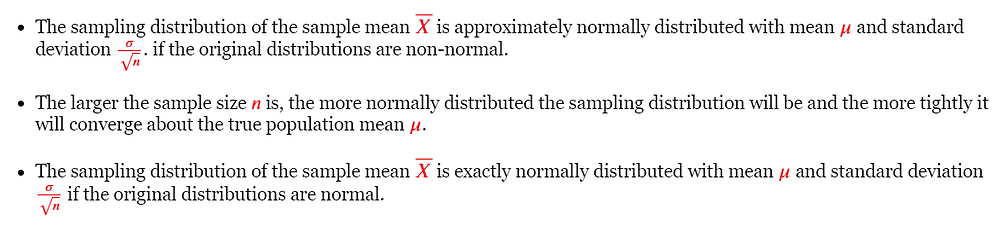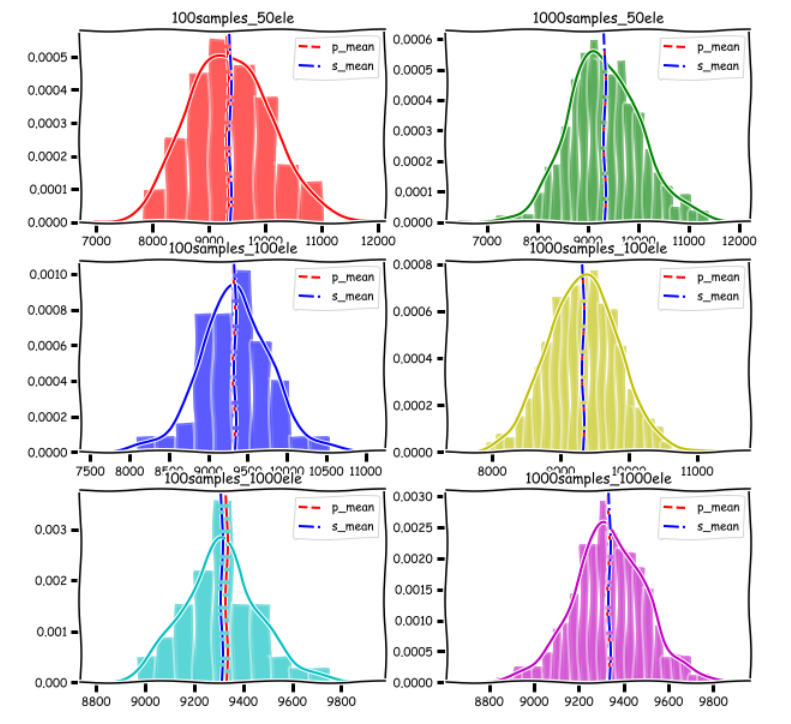Search

# Central Limit Theorem In Machine LearningCentral Limit theorem says:### Implementing Central Limit Theorm Using BlackFriday.csv Dataset

Import Libraries

```import pandas as pd
import numpy as np
import seaborn as sns
import matplotlib.pyplot as plt
import random
random.seed = 42
import warnings
warnings.filterwarnings("ignore")

import plotly.offline as offline
import plotly.graph_objs as go
offline.init_notebook_mode()
import pandas as pd
import matplotlib.pyplot as plt
from mpl_toolkits.mplot3d import Axes3D
from prettytable import PrettyTable
from IPython.display import HTML, display
plt.xkcd()

```

```# BlackFriday.csv
# The dataset here is a sample of the transactions made in a retail store on BlackFriday
# https://www.kaggle.com/mehdidag/black-friday

print("number of data points in our popultion:", df.shape)
print("% of missing values",df['Purchase'].isnull().sum() * 100 / len(df))

Output:

number of data points in our popultion: (537577, 12) % of missing values 0.0Find Mean and Standard Deviation of Population

```data = np.array(df['Purchase'].values)
print("Number of samples in our data: ",data.shape)
sns.distplot(data, color='g')
plt.show()

# population mean
population_mean = np.round(data.mean(),3)

# population std
population_std = np.round(data.std(),3)```

Output

Number of samples in our data: 537577Now Let's take 100 samples with each of size 100, and try to plot the distribution of their 'mean'

```def get_means_of_n_samples_with_m_size(data, n, m):
sample_mean_m_samples_n_ele = []
for i in range(0,n):
samples = random.sample(range(0, data.shape), m)
sample_mean_m_samples_n_ele.append(data[samples].mean())
return sample_mean_m_samples_n_ele```

Central Limit Theorm

```def central_limit_theorem(data, population_mean , i, j, color, key):
sns.distplot(np.array(data), color=color, ax=axs[i, j])
axs[i, j].axvline(population_mean, linestyle="--", color='r', label="p_mean")
axs[i, j].axvline(np.array(data).mean(), linestyle="-.", color='b', label="s_mean")
axs[i, j].set_title(key)
axs[i, j].legend()```

`sample_means = dict()`
```sample_means['100samples_50ele'] = get_means_of_n_samples_with_m_size(data,100, 50)
sample_means['1000samples_50ele'] = get_means_of_n_samples_with_m_size(data,1000, 50)

sample_means['100samples_100ele'] = get_means_of_n_samples_with_m_size(data,100, 100)
sample_means['1000samples_100ele'] = get_means_of_n_samples_with_m_size(data,1000, 100)

sample_means['100samples_1000ele'] = get_means_of_n_samples_with_m_size(data,100, 1000)
sample_means['1000samples_1000ele'] = get_means_of_n_samples_with_m_size(data,1000, 1000)```

Now Let's take 1000 samples with each of size 100, and try to plot the distribution of their 'mean'

```#red, green, blue, yellow, etc
colrs = ['r','g','b','y', 'c', 'm', 'k']
plt_grid  = [(0,0), (0, 1), (1, 0), (1, 1), (2, 0), (2, 1)]
sample_sizes = [(100,50), (1000, 50), (100, 100), (1000, 100), (100, 1000), (100, 1000)]

fig, axs = plt.subplots(3, 2,  figsize=(10, 10))
for i, key in enumerate(sample_means.keys()):
central_limit_theorem(sample_means[key], population_mean , plt_grid[i], plt_grid[i] , colrs[i], key)
plt.show()```

Outputif we can observe the thrid row distribution plots, the larger the sample size, the more it looks like Gaussian

Note : For the Central limit theorem to be valid, the samples have to be reasonably large. How large is that? It depends on how far the population distribution differs from a Gaussian distribution. Assuming the population doesn't have a really unusual distribution, a sample size of 10 or so is generally enough to invoke the Central Limit Theorem.

__ Let us get the properties of these sample distributions and compare these stats with the original distribution__

```x = PrettyTable()
x = PrettyTable(["#samples_name", "P_Mean", "Sampel mean", "P_Std", "Sample Std", "mu_x"+u"\u2248"+"mu", "std_x"+u"\u2248"+"std/"+u"\u221A"+"n"])

for i, key in enumerate(sample_means.keys()):
sample_mean = np.round(np.array(sample_means[key]).mean(), 3)
sample_std = np.round(np.array(sample_means[key]).std(), 3)
population_std_est = np.round(population_std/np.sqrt(sample_sizes[i]), 3)

row = []
row.append(key)
row.append(population_mean)
row.append(sample_mean)
row.append(population_std)
row.append(sample_std)
row.append(str(sample_mean)+u"\u2248"+str(population_mean))
row.append(str(sample_std)+u"\u2248"+str(population_std_est))
print(x)

```

OutputSend your request at realcode4you@gmail.com and get instant help with an affordable price.

We are always focus to delivered unique or without plagiarism code which is written by our highly educated professional which provide well structured code within your given time frame.

If you are looking other programming language help like C, C++, Java, Python, PHP, Asp.Net, NodeJs, ReactJs, etc. with the different types of databases like MySQL, MongoDB, SQL Server, Oracle, etc. then also contact us.